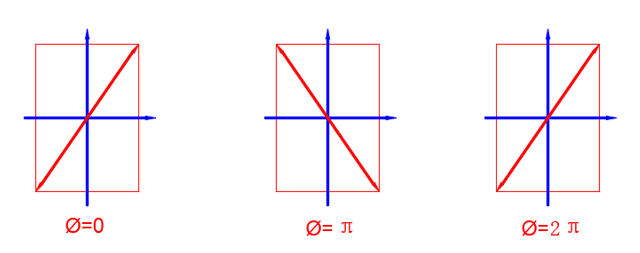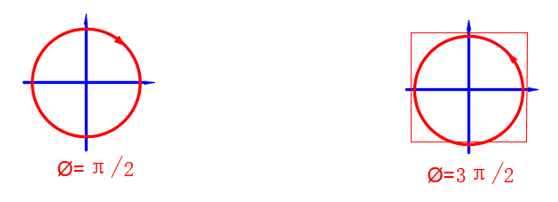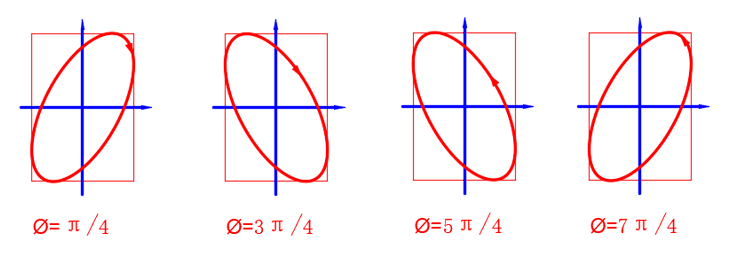## Lesson 12 – Polarization Revisited

Posted on: Monday, June 6th, 2022  In: Learning Optics with Austin

Now that we have explored light waves, we can dive more in depth into polarization. In how to describe light, we mentioned that sunlight is a form of unpolarized light or natural light. But what exactly causes light to become polarized? As light is an electromagnetic wave, it has both an electric field and magnetic field. The polarization state is defined by the electric field which can be linear, elliptical or circular.“Linear Polarization

Linear polarized light travels in a wave perpendicular to the direction of propagation. This wave looks like a line when viewed from the perspective of the light coming at you. The light oscillates along this line in a wave. For circular polarized light, light travels… you guessed it- in a circular fashion. Depending on whether the light travels clockwise or counter-clockwise, we label the polarization as right-hand circular polarization or left-hand circular polarization respectively. For elliptical polarization, the same naming convention goes except for light traveling in an elliptical, rather than circular fashion.Circular Polarization

A simple example again goes back to the 3D glasses in movies. If you pop out one of the lenses and hold it to a computer screen, you will see that turning the lens 90 degrees will either make the lens opaque or transparent to the computer light. This is because unlike sunlight, the light from a computer is polarized. Hence, holding a polarized lens at different angles changes the polarized states that are able to pass through.Elliptical Polarization

If natural light hits a surface and reflects, it becomes linearly polarized light. Natural light that is refracted becomes partially polarized light. Assuming that the intensity of the original unpolarized light is I0, the linearly polarized output would have an intensity of 50% of the original, or I1 = 0.5I0. If the linearly polarized light passes through a polarizer again, the intensity of the output (I2) is equivalent to I1 cos2(α).

Next: Lesson 13 – Diffraction Grating and Wave Plates